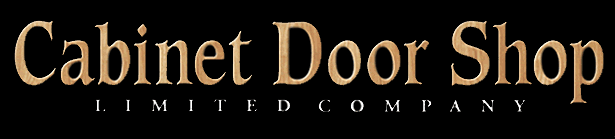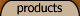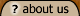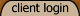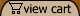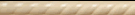# Thank you for selecting Cabinet Door Shop America's Cabinet Door Manufacturer! <!-- var aPC = new Array(); var isW3 = (document.getElementById&&true); var tax=0; function dummyfunc(){}; aPC=8.01;aPC=8.01;aPC=0;aPC=0;aPC=0;aPC=0;aPC=0;aPC=0; function pricechecker(i){ if(i!='') return(aPC[i]); return(0); } function enterValue(theObj){ alert('Please enter a value where indicated.'); theObj.focus(); return(false); } function checkStock(theObj,i){ if(i!='' && aPS[i] > 0) return(true); alert('I\'m sorry, that particular option is currently out of stock'); theObj.focus(); return(false); } function chooseOption(theObj){ alert('Please choose from the available product options.'); theObj.focus(); return(false); } function dataLimit(theObj){ alert('Please enter a maximum of 255 chars in the field indicated.'); theObj.focus(); return(false); } function formatprice(i, currcode, currformat){ var pTemplate='\$0.00'; if(currcode!='') pTemplate=' 0.00' + (currcode!=' '?'<b>'+currcode+'</b>':''); if(currcode==' JPY') i = Math.round(i).toString(); else if(i==Math.round(i)) i=i.toString()+".00"; else if(i*10.0==Math.round(i*10.0)) i=i.toString()+"0"; else if(i*100.0==Math.round(i*100.0)) i=i.toString(); if(currcode!="")pTemplate = currformat.toString().replace(/%s/,i.toString());else pTemplate = pTemplate.toString().replace(/\d[,.]*\d*/,i.toString());return(pTemplate);} //--> <!-- function formvalidator0(theForm) { if(theForm.optn0.selectedIndex==0)return(chooseOption(theForm.optn0)); if(theForm.voptn1.value=='')return(enterValue(theForm.voptn1)); if(theForm.voptn1.value.length>255)return(dataLimit(theForm.voptn1)); if(theForm.voptn2.value=='')return(enterValue(theForm.voptn2)); if(theForm.voptn2.value.length>255)return(dataLimit(theForm.voptn2)); return (true); } function openEFWindow(id) { popupWin = window.open('emailfriend.php?id='+id,'email_friend','menubar=no, scrollbars=no, width=400, height=400, directories=no,location=no,resizable=yes,status=no,toolbar=no') } //--> Home » Drawer Fronts & Dovetail Drawer Boxes (Order/Quote Form accessible from left side menu) » Uniboard Drawer FrontsRouted Solid SlabRouted Solid Slab Drawer Front One Piece Routed Double Refined Fiberboard Paint Grade Material Edge Profile: Please Select...SEI MJ CJ NR BB MACHINE Width: Height:   You can add this item to your quote or order.  « Previous | Next »

Copyright 2008 Cabinet Door Shop Limited Company